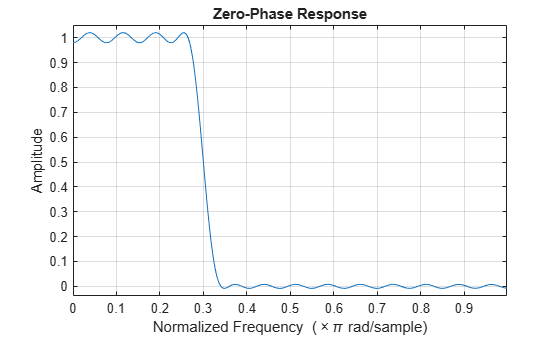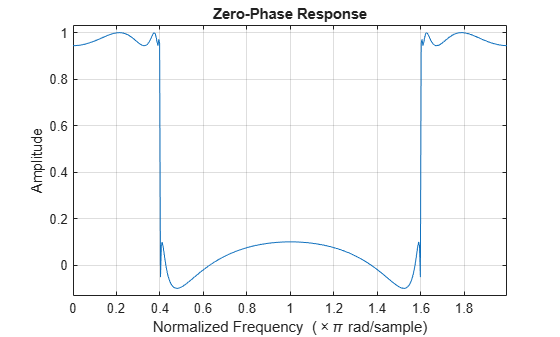# zerophase

Zero-phase response of digital filter

## Syntax

``[Hr,w] = zerophase(b,a)``
``[Hr,w] = zerophase(sos)``
``[Hr,w] = zerophase(d)``
``[Hr,w] = zerophase(___,nfft)``
``[Hr,w] = zerophase(___,nfft,"whole")``
``[Hr,f] = zerophase(___,fs)``
``[Hr,f] = zerophase(___,"whole",fs)``
``Hr = zerophase(___,w)``
``Hr = zerophase(___,f,fs)``
``[Hr,w,phi] = zerophase(___)``
``zerophase(___)``

## Description

example

````[Hr,w] = zerophase(b,a)` returns the zero-phase response `Hr` and the angular frequencies `w` at which the zero-phase response is computed, given a filter defined by numerator coefficients `b` and denominator coefficients `a`. The function evaluates the zero-phase response at `512` equally spaced points on the upper half of the unit circle.```
````[Hr,w] = zerophase(sos)` returns the zero-phase response for the second order sections matrix `sos`.```

example

````[Hr,w] = zerophase(d)` returns the zero-phase response for the digital filter `d`. Use `designfilt` to generate `d` based on frequency-response specifications.```

example

````[Hr,w] = zerophase(___,nfft)` uses `nfft` frequency points on the upper half of the unit circle to compute the zero-phase response. You can use an input combination from any of the previous syntaxes.```

example

````[Hr,w] = zerophase(___,nfft,"whole")` uses `nfft` frequency points around the whole unit circle to compute the zero-phase response.```

example

````[Hr,f] = zerophase(___,fs)` uses the sample rate `fs` to determine the frequencies `f` at which the zero-phase response is computed.```

example

````[Hr,f] = zerophase(___,"whole",fs)` uses the sample rate `fs` to determine the frequencies `f` around the whole unit circle at which the zero-phase response is computed.```
````Hr = zerophase(___,w)` returns the zero-phase response `Hr` evaluated at the angular frequencies specified in `w`.```
````Hr = zerophase(___,f,fs)` returns the zero-phase response `Hr` evaluated at the frequencies specified in `f`.```
````[Hr,w,phi] = zerophase(___)` returns the zero-phase response `Hr`, angular frequencies `w`, and continuous phase component `phi`.```
````zerophase(___)` plots the zero-phase response versus frequency. If you input the filter coefficients or second order sections matrix, the function plots in the current figure window. If you input a `digitalFilter`, the function displays the step response in FVTool. NoteIf the input to `zerophase` is single precision, the function calculates the zero-phase response using single-precision arithmetic. The output `Hr` is single precision. ```

## Examples

collapse all

Use `designfilt` to design a 54th-order FIR filter with a normalized cutoff frequency of 0.3π rad/sample, a passband ripple of 0.7 dB, and a stopband attenuation of 42 dB. Use the method of constrained least squares. Display the zero-phase response.

```Nf = 54; Fc = 0.3; Ap = 0.7; As = 42; d = designfilt("lowpassfir",FilterOrder=Nf,CutoffFrequency=Fc, ... PassbandRipple=Ap,StopbandAttenuation=As,DesignMethod="cls"); zerophase(d)```Design the same filter using `fircls1`, which uses linear units to measure the ripple and attenuation. Display the zero-phase response.

```pAp = 10^(Ap/40); Apl = (pAp-1)/(pAp+1); pAs = 10^(As/20); Asl = 1/pAs; b = fircls1(Nf,Fc,Apl,Asl); zerophase(b)```Design a 10th-order elliptic lowpass IIR filter with a normalized passband frequency of 0.4π rad/sample, a passband ripple of 0.5 dB, and a stopband attenuation of 20 dB. Display the zero-phase response of the filter on 512 frequency points around the whole unit circle.

```d = designfilt("lowpassiir",FilterOrder=10,PassbandFrequency=0.4, ... PassbandRipple=0.5,StopbandAttenuation=20,DesignMethod="ellip"); zerophase(d,512,"whole")```Create the same filter using `ellip`. Plot its zero-phase response.

```[b,a] = ellip(10,0.5,20,0.4); zerophase(b,a,512,"whole")```## Input Arguments

collapse all

Filter coefficients, specified as vectors. For FIR filters where `a=1`, you can omit the value `a` from the command.

Data Types: `single` | `double`

Second order sections, specified as a k-by-6 matrix where the number of sections k must be greater than or equal to 2. If the number of sections is less than 2, the function considers the input to be the numerator vector `b`. Each row of `sos` corresponds to the coefficients of a second order (biquad) filter. The ith row of the `sos` matrix corresponds to `[bi(1) bi(2) bi(3) ai(1) ai(2) ai(3)]`.

Data Types: `single` | `double`

Digital filter, specified as a `digitalFilter` object.

Number of frequency points used to compute the zero-phase response, specified as a positive integer scalar. For best results, set `nfft` to a value greater than the filter order.

Data Types: `double`

Angular frequencies at which the zero-phase response is computed, specified as a vector and expressed in radians/sample. `w` must have at least two elements.

Data Types: `double`

Frequencies at which the zero-phase response is computed, specified as a vector and expressed in hertz. `f` must have at least two elements.

Data Types: `double`

Sample rate, specified as a positive scalar.

Data Types: `double`

## Output Arguments

collapse all

Zero-phase response, returned as a vector.

The zero-phase response, Hr(ω), is related to the frequency response, H(e), by

`$H\left({e}^{j\omega }\right)={H}_{r}\left(\omega \right){e}^{j\phi \left(\omega \right)},$`

where φ(ω) is the continuous phase.

Note

The zero-phase response is always real, but it is not the equivalent of the magnitude response. The former can be negative while the latter cannot be negative.

Angular frequencies at which the zero-phase response is computed, returned as a vector.

Frequencies at which the zero-phase response is computed, returned as a vector.

Continuous phase component, returned as a vector. This quantity is not equivalent to the phase response of the filter when the zero-phase response is negative.

 Antoniou, Andreas. Digital Filters. New York: McGraw-Hill, Inc., 1993.